# Negative feedback stability

paulmdrdo
TL;DR Summary
Trying to make sense of the stability of a negative feedback.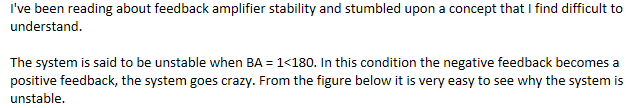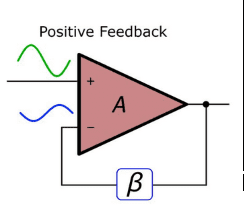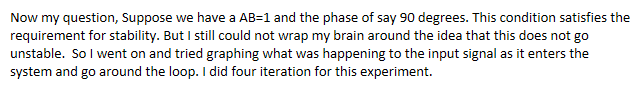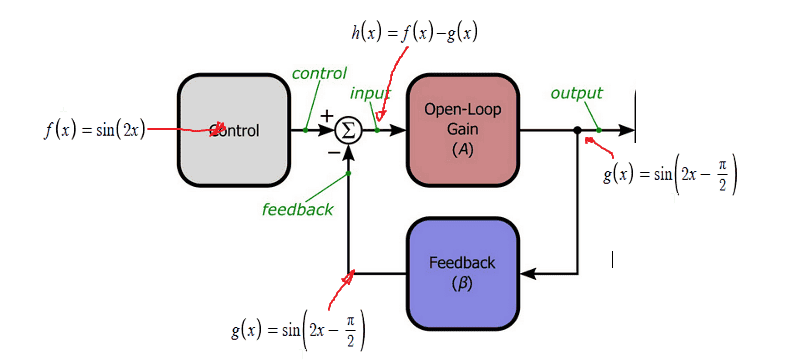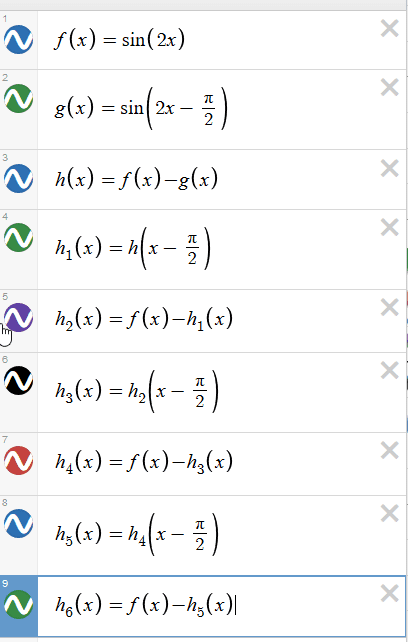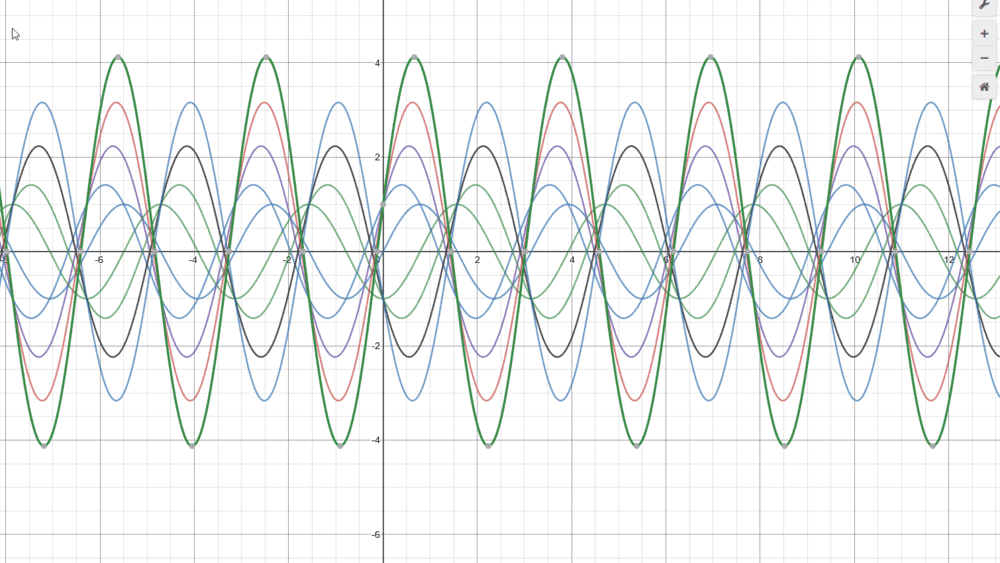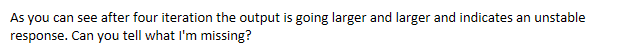#### Attachments

LvW
Most important: Show the following three functions in one diagram: f(x), g(x) and h(x)=f(x)-g(x),

•berkeman
paulmdrdo
Most important: Show the following three functions in one diagram: f(x), g(x) and h(x)=f(x)-g(x),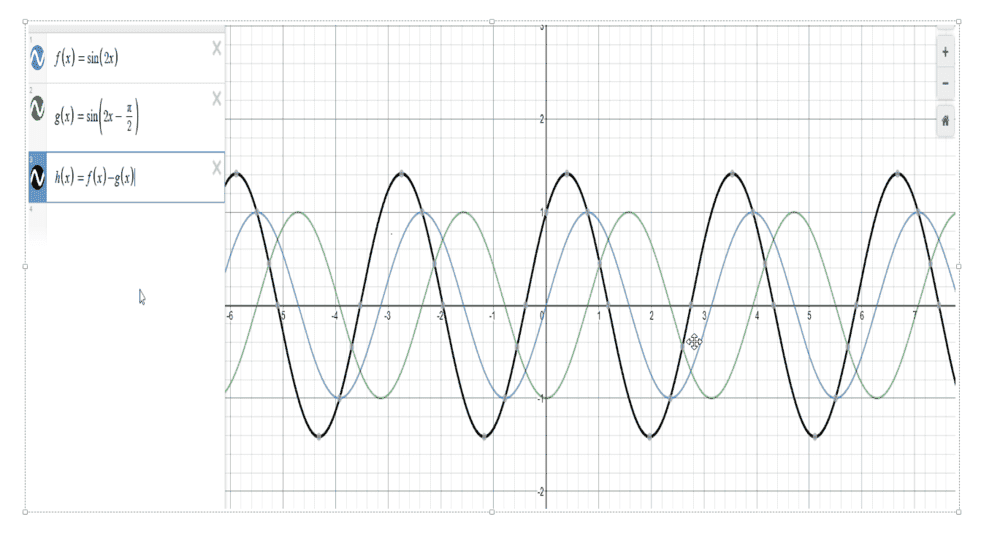willem2
Starting with the calculation for h1 you actually apply a phase lag of 180 degrees. The period of the signal is π so h(x-π/2) will lag h(x) by half a period.

paulmdrdo
Starting with the calculation for h1 you actually apply a phase lag of 180 degrees. The period of the signal is π so h(x-π/2) will lag h(x) by half a period.
What is the proper way of doing it then? Isn't the difference f(x)-g(x) supposed to be delayed by 90 deg when it passes the loop?

The open loop gain term A does not appear in your equations.
The open loop gain of the amplifier should be very high.
The loss and the phase shift in the feedback loop should be low for low frequencies.
The amplifier is an op-amp, the output changes, hopefully to make the inputs equal.

The feedback gain above the frequency where 180° phase shift occurs must be less than 1/A. You should only test with frequencies below that frequency.

Last edited:
willem2
What is the proper way of doing it then? Isn't the difference f(x)-g(x) supposed to be delayed by 90 deg when it passes the loop?
If you want that, you should use a delay of a quarter of the full period, so you must use h1(x) = h(x-π/4). Your calculation of g(x) is still correct because sin (2x - π/2) = sin (2 (x-π/4)) so you have the correct delay there.

Gold Member
Hi Paul;

This approach uses a circuit simulator such as the free LTSpice. The advantage of such a simulator is that it uses circuit components and automatically handles the appropriate equations based on the components used. Hopefully this will give you enough of a handle on the situation that you can (eventually) write the equations directly and do a straight mathematical simulation.

I suggest the following:
1. Start with a frequency low enough that any phase shifts do not interfere with operation
2. Get the circuit working without feedback, signal to (+) input, (-) input thru a 10k resistor to Ground. Define the amplifier gain as G=2
3. The amplifier output should be +2f(x), that is twice the input amplitude
4. Now implement the feedback circuit β with a gain of 0.5. Connect a 10k resistor between the amplifier output and the (-) input
5. The amplifier output should be 1.33f(x)
• Note: the 1.33f(x) output rather than the expected 2.0f(x) output is because of the low amplifier gain
6. Increase the amplifier gain, G, to 1,000,000
7. The amplifier output should be 2.0f(x)
Once the circuit is working as above, you can start playing with the gain, or frequency response and phase shifts to see what happens.

To change the gain, change the 10k resistor from (-) input to ground to a voltage divider. The amplifier (-) input goes to the tap of the divider. Try to keep the total divider resistance to ground around 10k, this makes it easier to play with the phase shift.

Probably the easiest way to change the phase shift is to change the 10k feedback resistor to three resistors in series, each of them 3.3k. Then connect a capacitor across each of the three resistors. The reason for three RC networks is to get 180° phase shift with three 60° shifts. If I got the calculation correct, at 1kHz about 30ηF should yield 180° phase shift. Capacitor values will scale inversely with frequency, i.e. use 3ηF for 10kHz. (Hmm... further thought says to double the capacitor values, oh well, try it and see what works)

Of course the drawback to all this is you have to learn a new program! LTSpice or similiar.

Hope this helps, it got more involved than I expected!

Cheers,
Tom

This approach uses a circuit simulator such as the free LTSpice. The advantage of such a simulator is that it uses circuit components and automatically handles the appropriate equations based on the components used.
One problem with Spice simulations is that the frequency response may look good, but the circuit will be unstable in the time domain. It is easy to get it wrong if it becomes non-linear.

An op-amp with an integrator (= 90° delay) in the feedback path, makes a high gain differentiator in the forward direction, not a stable amplifier. I would expect an output switching between high and low saturated limits.

Increase the amplifier gain, G, to 1,000,000
When the input signal is a unit phasor.
The feedback signal is the output phasor, rotated back, or delayed by 90°.
The question then becomes, what output phasor satisfies (+)input = (–)input.
The circuit can only be stable if the amplified differential input moves the output in a safe direction at each point in the cycle. You must solve for a stable output phasor.
I do not believe there is a stable solution.

LvW
Quote Baluncore: One problem with Spice simulations is that the frequency response may look good, but the circuit will be unstable in the time domain.

I want to restore confidence in Spice-based simulations. The problem is generally not the simulator, but in most cases the user, if he does not know exactly what to expect from the simulator.
In an AC analysis, an operating point is calculated for the given supply voltage, which is assumed to be ideal, constant and free of disturbances. Even a calculation by hand would - in case of an unstable arrangement - result in an apparently stable circuit.
This even applies to a positive DC feedback (by hand as well as simulation).
Only a TRAN analysis in the time domain with a supply voltage switched on at t=0 can reveal a case of instability.
I have been dealing with SPICE analyses for more than 20 years. In case of errors I was always the cause (errors in interpretation, evaluation, polarities, grounding, ...).

But there is an indication of instability in AC analysis: The phase response shows an abnormal (increasing) behaviour in the region of instability.

eq1
Summary:: Trying to make sense of the stability of a negative feedback

I don’t think the math in the image is correct which might be why you’re confused.

The output of the open gain amp should be Ah(x) but it is drawn as g(x) which means the f(x) term was dropped.

Also, one can definitely analyze this structure with math but to do this analysis you need to define what definition of “stability“ you’re using. There are options.

The usual solution is:

Ah(x)=g(x)
A(f(x)-Bg(x))=g(x)
Af(x)=(1+AB)g(x)
(1/(1/A)+B))f(x)=g(x), giving the output in terms of the input

In your example A and B are not equal to 1. They are an all pass filter with constant phase delay.

Last edited: